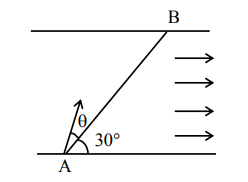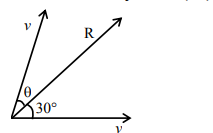# A swimmer wants to cross a river from point A to point B.`
Question:

A swimmer wants to cross a river from point A to point B. Line AB makes an angle of 30° with the flow of river. Magnitude of velocity of the swimmer is same as that of the river. The angle  with the line AB should be ____°, so that the swimmer reaches point B.Solution:Both velocity vectors are of same magnitude therefore resultant would pass exactly midway through them

$\theta=30^{\circ}$